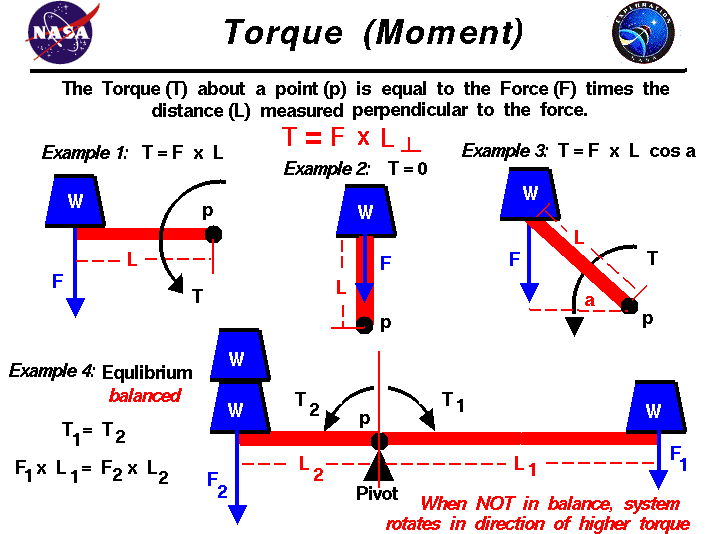+ Text Only Site
+ Non-Flash Version
+ Contact GlennA force may be thought of as a push or pull in a specific direction. When a force is applied to an object, the resulting motion of the object depends on where the force is applied and how the object is confined. If the object is unconfined and the force is applied through the center of gravity, the object moves in pure translation, as described by Newton's laws of motion. If the object is confined (or pinned) at some location called a pivot, the object rotates about the pivot, but does not translate. The force is transmitted through the pivot and the details of the rotation depend on the distance from the applied force to the pivot. If the object is unconfined and the force is applied at some distance from the center of gravity, the object both translates and rotates about the center of gravity. The details of the rotation depend on the distance from the applied force to the center of gravity. The motion of flying objects and spacecraft is described by this third type of motion; a combination of translation and rotation. A force F is a vector quantity, which means that it has both a magnitude and a direction associated with it. The direction of the force is important because the translation of the object is in the same direction as the force. The product of the force and the perpendicular distance to the center of gravity for an unconfined object, or to the pivot for a confined object, is called the torque or the moment. A torque is also a vector quantity and produces a rotation in the same way that a force produces a translation. Namely, an object at rest, or rotating at a constant angular velocity, will continue to do so until it is subject to an external torque. A torque produces a change in angular velocity which is called an angular acceleration. On the figure, we show four examples of torques to illustrate the basic principles governing torques. In the examples, we will study the action of torques on a confined object. If the object were unconfined, the pivot p would be replaced by the center of gravity cg. In each example a blue weight W generates a force F on a red bar, which is called an arm. The distance L used to determine the torque T is the distance from the pivot to the force, but measured perpendicular to the direction of the force. In Example 1, the force is applied perpendicular to the arm. In this case, the perpendicular distance is the length of the bar and the torque is equal to the product of the length and the force. T = F * L In Example 2, the same force is applied to the arm, but the force now acts right through the pivot. In this case, the distance from the pivot perpendicular to the force is zero. So, in this case: T = 0 Think of a hinged door. If you push on the edge of the door, towards the hinge, the door doesn't move because the torque is zero. Example 3 is the general case in which the force is applied at some angle a to the arm. The perpendicular distance is given by trigonometry as the length of the arm (L) times the cosine (cos) of the angle. The torque is then given by: T = F * L * cos(a) Examples 1 and 2 can be derived from this general formula, since the cosine of 0 degrees is 1.0 (Example 1), and the cosine of 90 degrees is 0.0 (Example 2). In Example 4, the pivot has been moved from the end of the bar to a location near the middle of the bar. Weights are added to both sides of the pivot. To the right a single weight W produces a force F1 acting at a distance L1 from the pivot. This creates a torque T1 equal to the product of the force and the distance. T1 = F1 * L1 To the left of the pivot two weights W produce a force F2 at a distance L2. This produces a torque T2 in a direction opposite from T1 because the distance is in the opposite direction. T2 = F2 * L2 If the system were in equilibrium, or balanced, the torques would be equal and no net torque would act on the system. T1 = T2 or T1 - T2 = 0 F1 * L1 = F2 * L2 If the system is not in equilibrium, or unbalanced, the bar rotates about the pivot in the direction of the higher torque. If F2 = 2 * F1, what is the relation between L1 and L2 to balance the system? If F2 = 2 * F1, and L1 = L2, in which direction would the system rotate? Aerospace engineers use torques to stabilize and control flying objects. On airplanes, the control surfaces produce aerodynamic forces which are applied at some distance from the center of gravity and therefore cause the aircraft to rotate. The same effect is used on rockets flying within the atmosphere. The aerodynamic forces act through the center of pressure to provide stability during powered flight. On most full scale rockets, the thrust of the nozzle is gimbaled, or angled, to produce a torque to maneuver the rocket in flight. In orbit, small thrusters can torque the spacecraft to point in a desired direction. Guided ToursForces, Torques and Motion:Rocket Rotation:Guidance System:Activities: Related Sites: Rocket Index Rocket Home Beginner's Guide Home+ Inspector General Hotline + Equal Employment Opportunity Data Posted Pursuant to the No Fear Act + Budgets, Strategic Plans and Accountability Reports + Freedom of Information Act + The President's Management Agenda + NASA Privacy Statement, Disclaimer, and Accessibility CertificationEditor: Tom Benson NASA Official: Tom Benson Last Updated: May 13 2021 + Contact Glenn